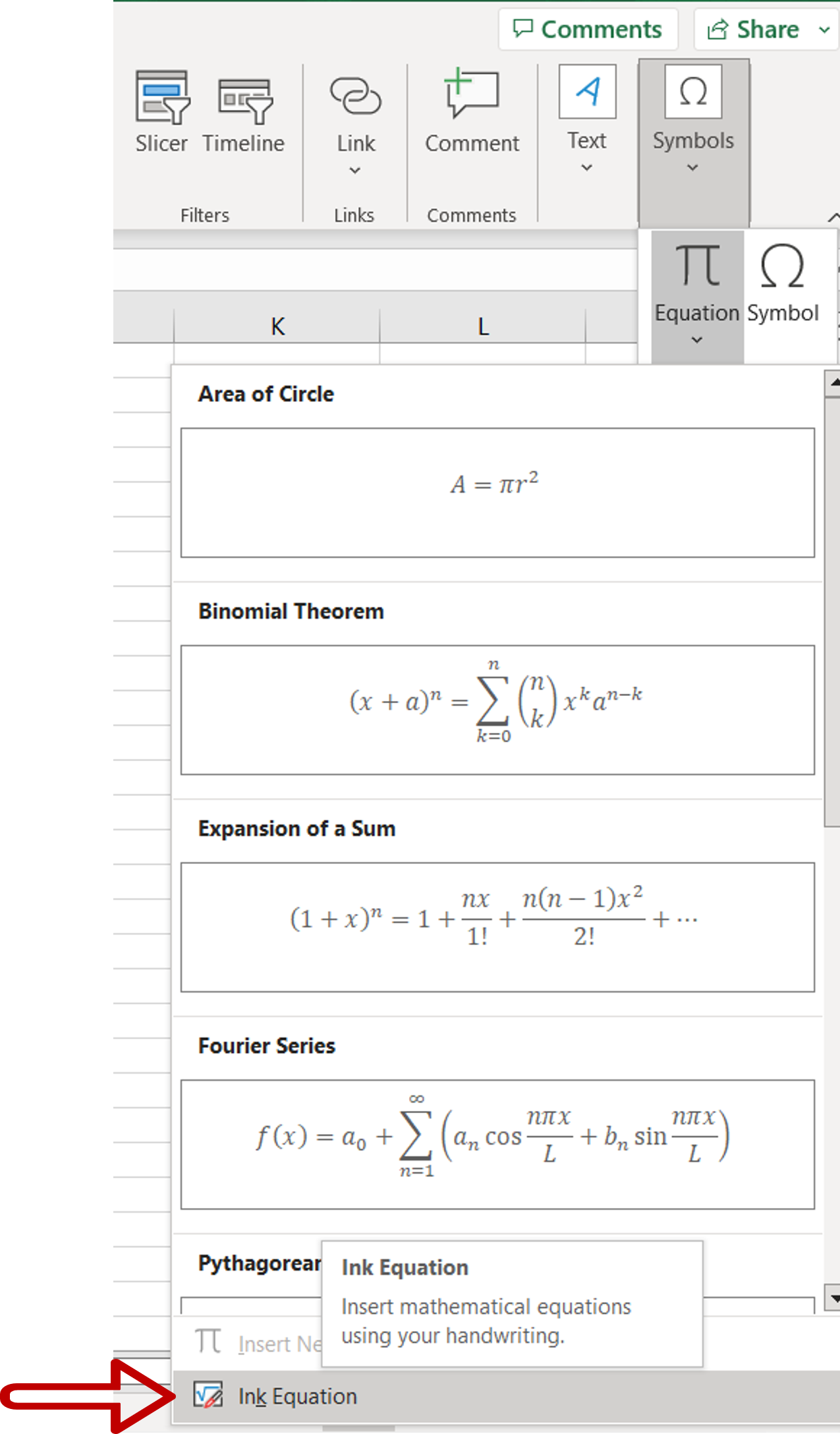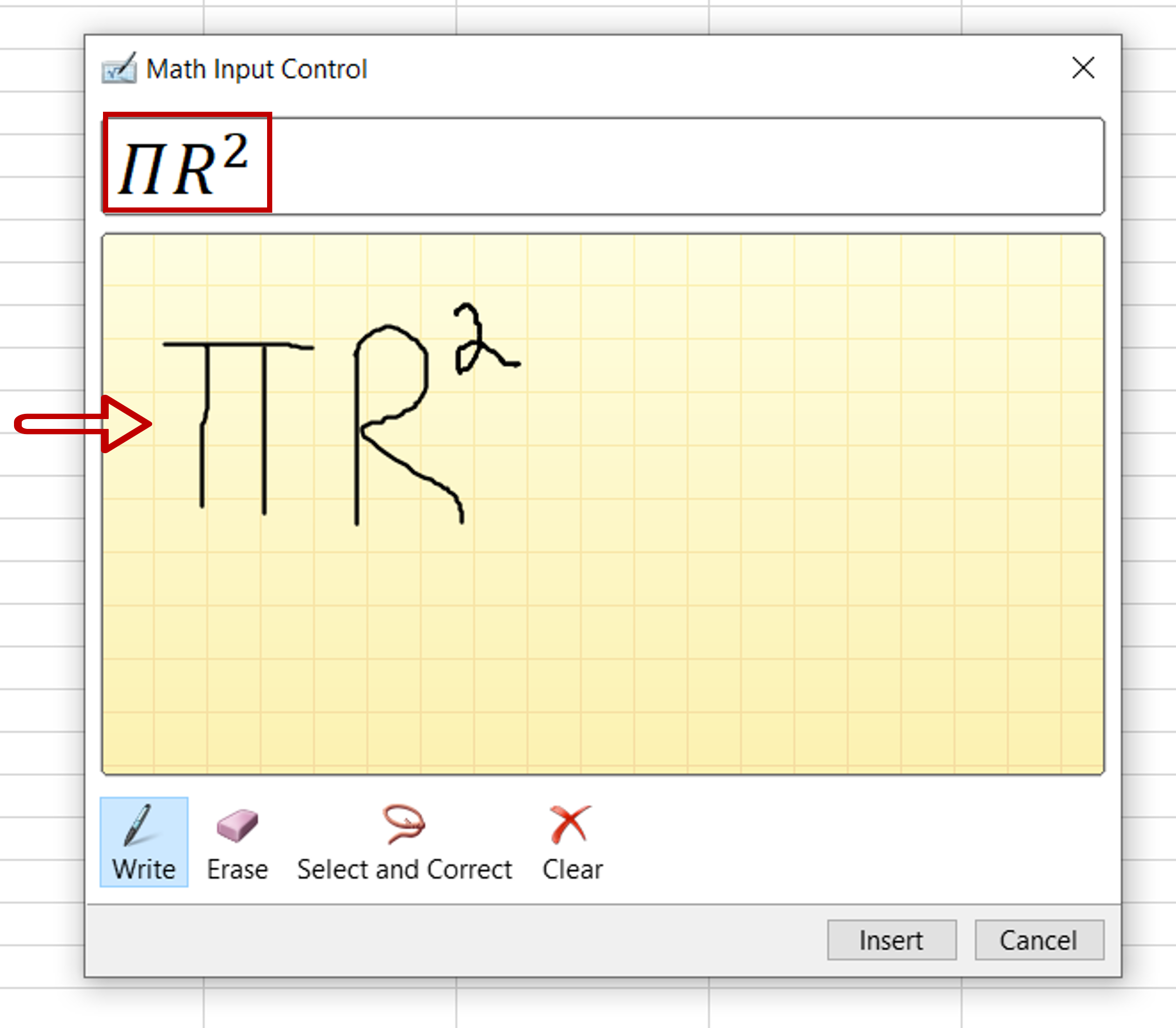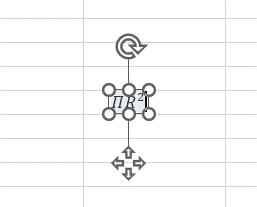# How to write an equation in Excel

You can watch a video tutorial here.Excel is primarily used for its spreadsheet functions but it also supports several textual functions.  When evaluating an equation, for example, the area of a circle, you can do so by typing the formula in the cell using the equal sign (=). The problem arises when you want to include the equation itself. It becomes a complex process to include the Pi character or numerators and denominators within the cell. To solve this problem, Excel has a tool in which you can write your own equation.

### Step 1 – Choose the menu option– Select any cell on the worksheet
– Go to Insert > Symbols
– Expand the Equation dropdown

### Step 2 – Open the Math Input Control box– Select Ink Equation

### Step 3 – Write the equation– Write the equation using the mouse
– Check the preview to see if the characters are displayed correctly
– Click Insert

### Step 4 – Check the result– The equation is inserted as an image